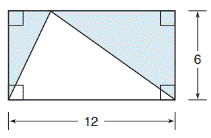Chapter 8.1, Problem 20EElementary Geometry For College St...

7th Edition
Alexander + 2 others
ISBN: 9781337614085

Solutions

Chapter
SectionElementary Geometry For College St...

7th Edition
Alexander + 2 others
ISBN: 9781337614085
Textbook Problem

In Exercises 19 to 22, find the area of the shaded region.To determine

To find:

The area of the shaded region.

Explanation

Finding the area of the figure is followed by identifying the figure and find area with the suitable geometry formulae.

Calculation:

Given,

Which represents the triangle inscribed in a rectangle.

The area of the shaded region(i.e.) A=lw12(bh)}=(A1)Area of a rectangle-(A2)Area of a triangle

Here,

Length of the rectangle (l)=12

Width of the rectangle (w)=6

Still sussing out bartleby?

Check out a sample textbook solution.

See a sample solution

The Solution to Your Study Problems

Bartleby provides explanations to thousands of textbook problems written by our experts, many with advanced degrees!

Get Started

Find more solutions based on key concepts